# Krull intersection theorem for Noetherian domains

This article defines a result where the base ring (or one or more of the rings involved) is Noetherian
View more results involving Noetherianness or Read a survey article on applying Noetherianness
This fact is an application of the following pivotal fact/result/idea: Krull intersection theorem
View other applications of Krull intersection theorem OR Read a survey article on applying Krull intersection theorem

## Statement

Let$R$ be a Noetherian domain (i.e. a Noetherian ring that is also an integral domain) and$I$ a proper ideal in$R$. Then, we have: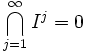$\bigcap_{j=1}^\infty I^j = 0$

## Proof

### Applying Krull intersection theorem for modules

The Krull intersection theorem states that if$M$ is a finitely generated module over a Noetherian ring$R$ and$I$ is an ideal inside$R$, then there exists$r \in I$ such that: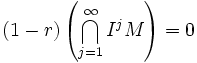$(1 - r)\left( \bigcap_{j=1}^\infty I^jM \right) = 0$

We apply this to the case where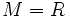$M = R$, to get that there exists$r \in I$, such that: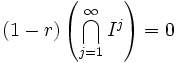$(1 - r)\left( \bigcap_{j=1}^\infty I^j\right)=0$

### Applying the integral domain condition and properness of the ideal

Since$I$ is a proper ideal,$1 \notin I$. Hence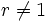$r \ne 1$, so the element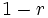$1 - r$ cannot be zero.

Thus, by the fact that we are in an integral domain, and by the above equation, we get:$\bigcap_{j=1}^\infty I^j = 0$